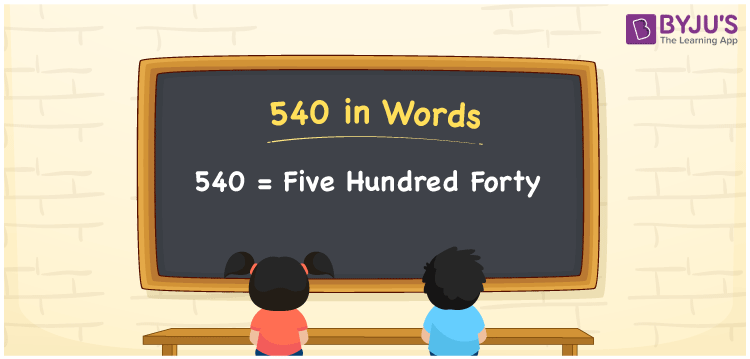# 540 in Words

540 in words is written as Five hundred forty. In both the International System of Numerals and the Indian System of Numerals, 540 is written as Five hundred forty. The number 540 is a Cardinal Number as it represents some quantity. For example, “the cost of notebooks is 540 rupees”.

 540 in Words Five hundred forty Five hundred forty in Number 540

## 540 in English Words

We write 540 in English Words using the letters of the English alphabet. Therefore, we read 540 in English as “Five hundred forty.”## How to Write 540 in Words?

To write 540 in words, we shall use the place value chart. In the place value chart, write 0 in the ones, 4 in the tens, and 5 in the hundreds. Now let us make a place value chart to write the number 540 in words.

 Hundreds Tens Ones 5 4 0

Thus, we can write the expanded form as

5 × Hundred + 4 × Ten + 0 × One

= 5 × 100 + 4 × 10 + 0 × 1

= 500 + 40 + 0

= 540

= Five Hundred Forty

540 is a natural number which is the successor of 539 and the predecessor of 541.

540 in words – Five Hundred Forty

• Is 540 an odd number? – No
• Is 540 an even number? – Yes
• Is 540 a perfect square number? – No
• Is 540 a perfect cube number? – No
• Is 540 a prime number? – No
• Is 540 a composite number? – Yes

## Frequently Asked Questions on 540 in Words

Q1

### How to write 540 in words?

540 in words is written as Five Hundred Forty.
Q2

### How to write 540 in the International and Indian System of Numerals?

In both, the system of numerals, 540 in words, is written as Five Hundred Forty.
Q3

### How to write 540 in a place value chart?

In the place value chart, write 0 in the ones, 4 in the tens, and 5 in the hundreds.